### Reverse String - keeping performance in mind (without StringBuffer) in java

In this programming tutorial we will learn how to reverse String in java with example, diagram and program, and we will keep performance in mind and without using without StringBuffer in java.

Complexity offered by our program to reverse String in java will be O(n/2), where n is number of characters in string in java.

Method used to reverse String in java example - reverseString()

Logic explanation to reverse String in java with diagram1 >

Initially String is =abcde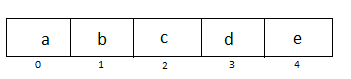STEP1>
i=0, j=4 swap them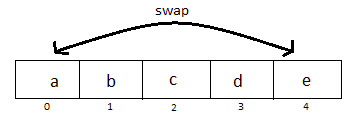STEP2>
i=1,j=3 swap them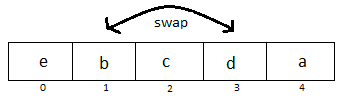Reversed=edcba.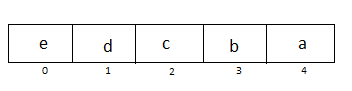Logic explanation to reverse String in java with diagram2 >

Initially String is =abcd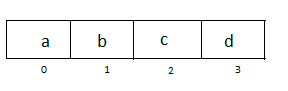STEP1>
i=0, j=3 swap them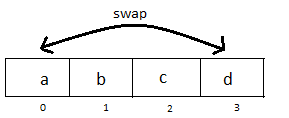STEP2>
i=1,j=2 swap them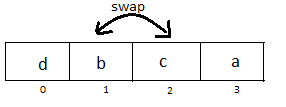Reversed=dcba.Example1 (dry run)> Reverse string
abcde >  edcba

Our initial array is

STEP1: i=0, j=4 (swap both positions)
STEP2: i=1, j=3 (swap both positions)
Now, i=2, we will exit for loop and our string is reversed.

Example2 (dry run)> Reverse string
abcd >  dcba

Our initial array is

STEP1: i=0, j=3 (swap both positions)
STEP2: i=1, j=2 (swap both positions)
Now, i=2, we will exit for loop and our string is reversed.

Complexity to reverse String in java without using StringBuffer in java >
Now, lets see how complexity is O(n/2).
We are executing only half the number of characters we have in our string.

Best case: O(n/2)- (1/2), when we have odd number of characters in string.
Average case: O(n/2) , generally when we have even number of characters in string.
Worst case: O(n/2).

Full Program/SourceCode/Example to reverse String in java without using StringBuffer in java >
 /** Copyright (c), AnkitMittal www.JavaMadeSoEasy.com */ public class ReverseStringExample {    public static void main(String...args){           String originalString="abcde"; //String to be reversed                     System.out.println("Original String: "+originalString);           System.out.println("Reversed String: "+reverseString(originalString));            }       /*    * return reversed String.    */    public static String reverseString(String originalString){           char ar[]=originalString.toCharArray();           char temp;           for(int i=0,j=ar.length-1; i<(ar.length/2); i++,j--){                  temp=ar[i];                  ar[i]=ar[j];                  ar[j]=temp;           }           return new String(ar);    } } /*OUTPUT Original String: abcde Reversed String: edcba */

So in this programming tutorial we learned how to reverse String in java with example, diagram and program, and we will keep performance in mind and without using without StringBuffer in java.

Having any doubt? or you you liked the tutorial! Please comment in below section.